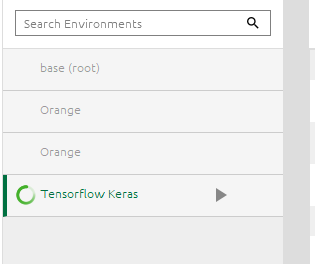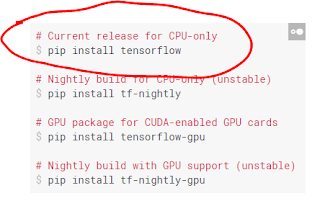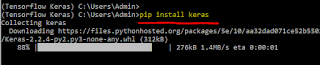# Data Science Simplified

Learning the Machine Learning, in a Human-friendly Way

### Building a Deep Learning Model using Keras

In this post, let us see how to build a deep learning model using Keras. If you haven't installed Tensorflow and Keras, I will show the simple way to install these two modules.

### 1. Installing Tensorflow and Keras

• Open Anaconda Navigator. Under Environments, create new environment in Python 3.6.Create a new environment in Anaconda- Python 3.6Created a new environment
•  Open terminal and pip install Tensorflow and KerasOpen TerminalUse the appropriate option for your computer (From Tensorflow website)

• pip install tensorflowinstall Tensorflow

• then, pip install kerasinstall Keras

• Now install Jupyter Notebook on this environment and launchNow install Jupyter Notebook and launch

• import tensorflow and kerasMission successful!

### 2. Building Model using Keras

#### 2.1 Four steps

Keras is simple to use. There are four steps in building a neural network model in Keras.
1. Define the architecture
2. Compile
3. Fit
4. Predict
In the following picture, I have shown these four steps (basic code is taken from here).A basic model using Keras in four steps

### 2.2 Four steps in detail

As you can see, first we have imported libraries.

#### a) Define the architecture

In sequential model, we can stack the layers. In the above example, we have added a hidden layer with 64 nodes and ReLU activation function. In addition, we also specify number of inputs or the input dimension (input_dim=20). If you are using input_shape then it will be (20,). And both denote the same thing. We have added output layer with 10 output nodes-one for each class of the output with softmax activation function as this is a multiple classification model.

#### b) Compile

Once the model has been specified, we need to compile the model. There are three arguments in compiling step:
1. Loss function,
2. Optimizer and
3. Metrics
b1) Loss function

In case of Loss function, commonly used are the
• mean_squared_error: commonly used for regression tasks
• mean_absolute_percentage_error
• categorical_crossentropy (Used for classification problems where y is one-hot-encoded, if not we can use to_categorical option to one-hot-encode y)

b2) Optimizer

Commonly used optimizers are
We can tune the learning rates, decay, momentum etc for better performance.

b3) Metrics

And in case metrics to measure the performance of the model, commonly used is the 'accuracy'

#### c) Fit

In the third step, we are fitting this model to the data. We can specify validation splits, epochs, early stopping monitor as shown below.

model.fit(X, target, validation_split=0.3, epochs=30, callbacks=[early_stopping_monitor])

#### d) Predict

And finally we are predicting the classes for new test data.

### Summary

In this post, we have seen

• how to install Tensorflow and Keras
• Four steps of basic model building in Keras
• Some important concepts/hyperparameters in those four steps

### 3. References

1. https://keras.io/ has all the resources to learn Keras.
2. For installing Tensorflow and Keras, refer https://towardsdatascience.com/python-environment-setup-for-deep-learning-on-windows-10-c373786e36d1
3. Also you may refer this page for installing Keras
4. Excellent blogpost series on training neural networks https://towardsdatascience.com/how-do-we-train-neural-networks-edd985562b73